# Decimal versus Binary Numeration

## Chapter 1 - Numeration Systems

Let’s count from zero to twenty using four different kinds of numeration systems: hash marks, Roman numerals, decimal, and binary: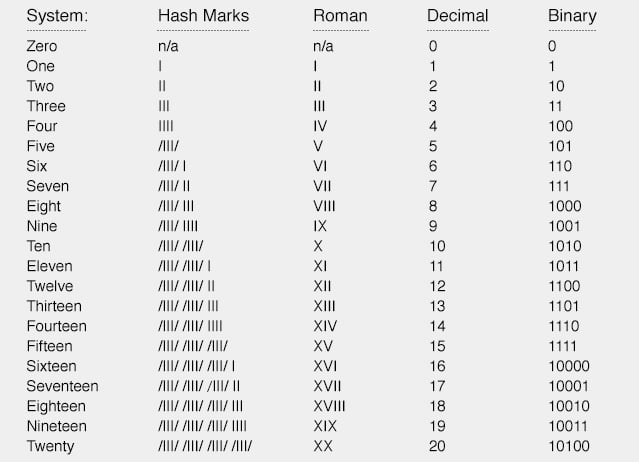Neither hash marks nor the Roman system are very practical for symbolizing large numbers. Obviously, place-weighted systems such as decimal and binary are more efficient for the task.

Notice, though, how much shorter decimal notation is over binary notation, for the same number of quantities. What takes five bits in binary notation only takes two digits in decimal notation.

This raises an interesting question regarding different numeration systems: how large of a number can be represented with a limited number of cipher positions, or places? With the crude hash-mark system, the number of places IS the largest number that can be represented, since one hash mark “place” is required for every integer step.

For place-weighted systems of numeration, however, the answer is found by taking base of the numeration system (10 for decimal, 2 for binary) and raising it to the power of the number of places.

For example, 5 digits in a decimal numeration system can represent 100,000 different integer number values, from 0 to 99,999 (10 to the 5th power = 100,000). 8 bits in a binary numeration system can represent 256 different integer number values, from 0 to 11111111 (binary), or 0 to 255 (decimal), because 2 to the 8th power equals 256.

With each additional place position to the number field, the capacity for representing numbers increases by a factor of the base (10 for decimal, 2 for binary).

An interesting footnote for this topic is the one of the first electronic digital computers, the Eniac.

The designers of the Eniac chose to represent numbers in decimal form, digitally, using a series of circuits called “ring counters” instead of just going with the binary numeration system, in an effort to minimize the number of circuits required to represent and calculate very large numbers.

This approach turned out to be counter-productive, and virtually all digital computers since then have been purely binary in design.

### Binary to Decimal Conversion

To convert a number in binary numeration to its equivalent in decimal form, all you have to do is calculate the sum of all the products of bits with their respective place-weight constants. To illustrate: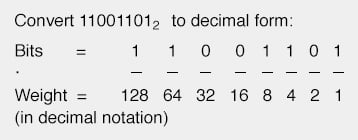The bit on the far right side is called the Least Significant Bit (LSB), because it stands in the place of the lowest weight (the one’s place).

The bit on the far left side is called the Most Significant Bit (MSB), because it stands in the place of the highest weight (the one hundred twenty-eight’s place).

Remember, a bit value of “1” means that the respective place weight gets added to the total value, and a bit value of “0” means that the respective place weight does not get added to the total value. With the above example, we have: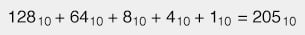If we encounter a binary number with a dot (.), called a “binary point” instead of a decimal point, we follow the same procedure, realizing that each place weight to the right of the point is one-half the value of the one to the left of it (just as each place weight to the right of a decimal point is one-tenth the weight of the one to the left of it). For example: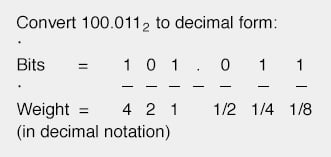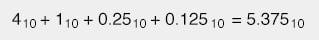RELATED WORKSHEETS:

• Share
Published under the terms and conditions of the Design Science License